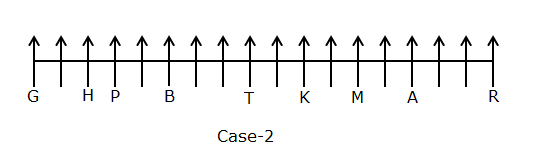# SBI Clerk Prelims Reasoning Ability Questions 2021 (Day-68)

Dear Aspirants, Our IBPS Guide team is providing new series of Reasoning Questions for SBI Clerk Prelims 2021 so the aspirants can practice it on a daily basis. These questions are framed by our skilled experts after understanding your needs thoroughly. Aspirants can practice these new series questions daily to familiarize with the exact exam pattern and make your preparation effective.

Start Quiz

Coding decoding

Direction (1-4): Study the following information carefully and answer the below questions.

In a certain code language

‘Students are attend class’ means ‘ef lg zt rm’,

‘They attend online class’ means ‘zt oe rm ps’,

‘Students hate online class’ means ‘ef zt oe ml’,

‘They are missing school’ means ‘ps kt rh lg’

1) What is the code for ‘attend’?

A.rm

B.zt

C.lg

D.ps

E.None of these

2) What is the code for ‘They Hate’?

A.rm oe

B.ml zt

C.zt oe

D.ef ml

E.ps ml

3) What does “zt” represent in the code language?

A.Students

B.Online

C.Attend

D.Class

E.None of the above

4) If “Missing” is coded as “rh” then Which of the following represents the code “kt oe lg”?

A.They attend online

B.Class are school

C.School are online

D.Students are school

E.None of the above

Misc

5) Four of the following five are alike in a certain way and form a group. Find the odd one out?

A.DH32

B.BK22

C.IC18

D.NA14

E.LE60

Unknown linear arrangement

Direction (6-10): Study the following information carefully and answer the questions given below it.

A certain number of persons are sitting in a row facing to the north direction. Eight persons are sitting between A and B. K sits fourth to the left of A. Four persons sit between B and G, who sits one of the extreme ends of the row. H sits third to the left of B. Two persons sit between G and P. R sits third to the right of A. The number of persons sits left of P is same as the number of persons sits right of A. Only one person sits between M and K. T sits fourth to the left of M. More than two persons sit between H and T.

6) How many seats are in the row?

A.17

B.18

C.19

D.20

E.None of these

7) How many known persons are sitting between M and R?

A.Five

B.Six

C.Eight

D.One

E.None of these

8) Who among the following sits second to the left of K?

A.A

B.M

C.T

D.H

E.None of these

9) How many known persons are sitting between H and K?

A.Six

B.Three

C.Nine

D.Eight

E.None of these

10) What is the position of P with respect to G?

A.Fourth right

B.Third left

C.Third right

D.Fourth left

E.None of these

Directions (1-4) :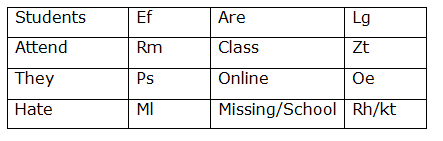The Number is representing the product of the place value of the first and second alphabet.

Ex: B(2)*K(11)=22

Directions (6-10) :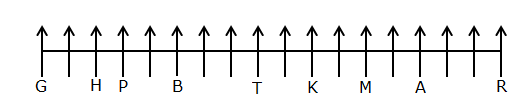Eight persons are sitting between A and B. K sits fourth to the left of A. Here, we have two cases.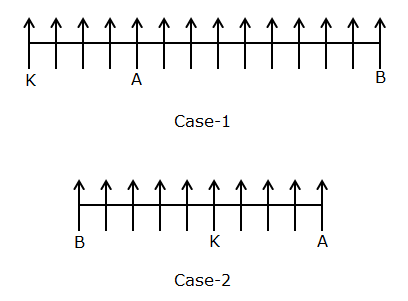Four persons sit between B and G, who sits one of the extreme ends of the row. H sits third to the left of B.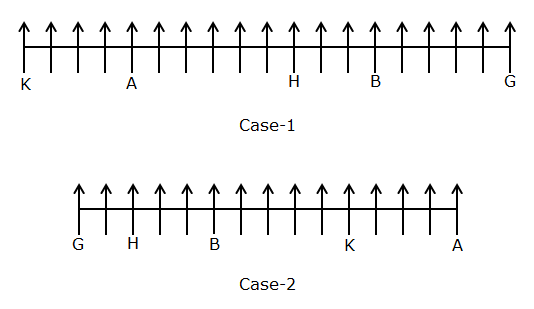Two persons sit between G and P. R sits third to the right of A.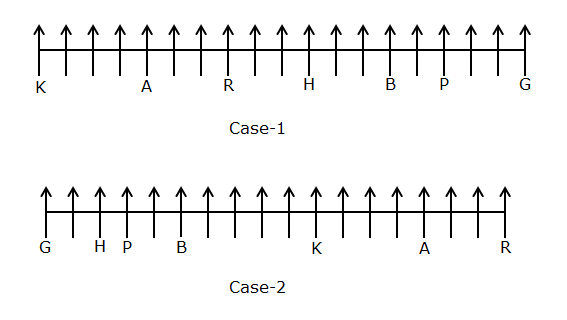The number of persons sits left of P is same as the number of persons sits right of A. Here, Case-1 is eliminated.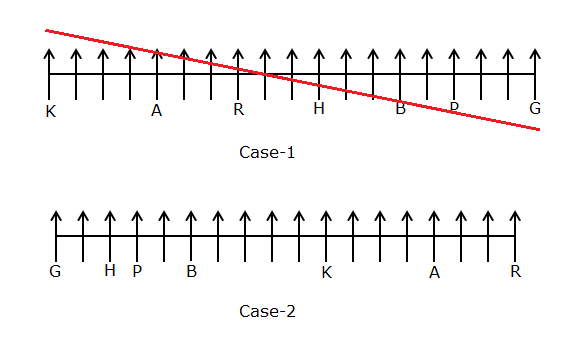Only one person sits between M and K. Here, we have one more case.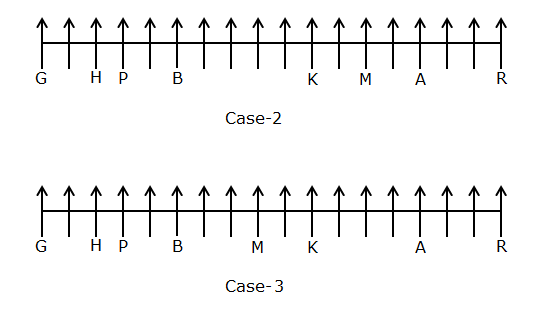T sits fourth to the left of M. More than two persons sit between H and T. Here, Case-3 is eliminated.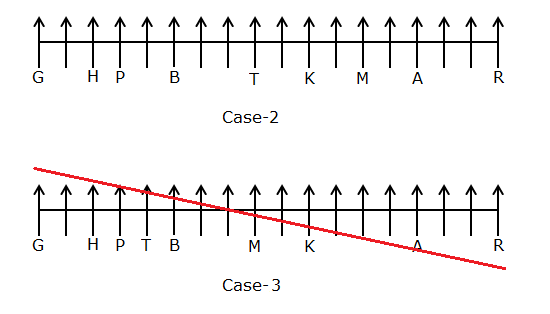Hence, final solution is Case-2.